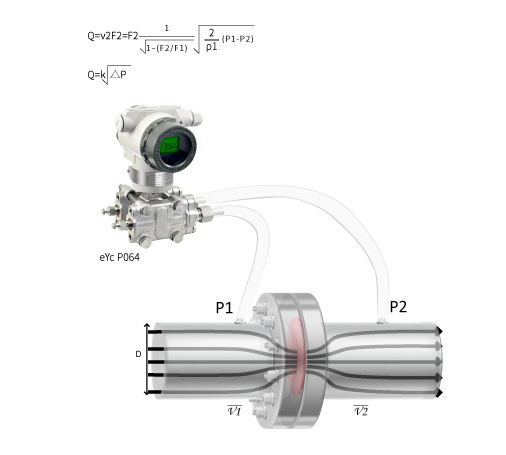## eyc-tech P064 Digital Differential Pressure Transmitter Principle Description

### When the fluid flow getting bigger, the pressure difference getting bigger at the same time, so the flow rate can be measured based on the pressure difference.

This measurement method is based on the Continuity equation(Law of conservation of mass) and the Bernoulli's principle(Conservation of energy) to achieve the purpose of accurate measurement.
As shown in the figure, the red part is the orifice plate installed in the pipeline, which is perpendicular to the flow direction.
Assuming that the fluid fills the pipeline, between the two-point cross section of side P1 and side P2 in the figure, it is according to the Continuity equatio(Law of conservation of mass) and the Bernoulli's principle(Conservation of energy), we can get formula (1) and formula (2)

ρ1ν12/2+P1=ρ1ν12/2+P2.......formula(1)
ρ1ν12F1=ρ1ν12F2..................formula(2)

ν：Average flow velocity(m/s)          P：Average flow rate(Pas abs)
ρ1：Fluid density(kg/m3)                   F：Fluid cross-sectional area(m3)

From formula(1)(2), the volume flow rate Q(m3/s) through the orifice plate is: